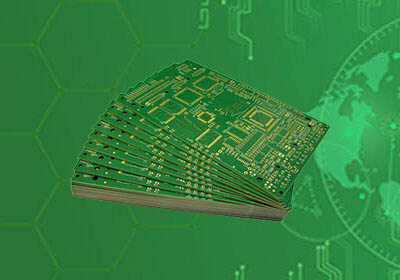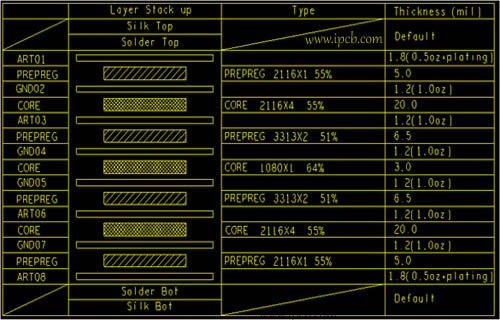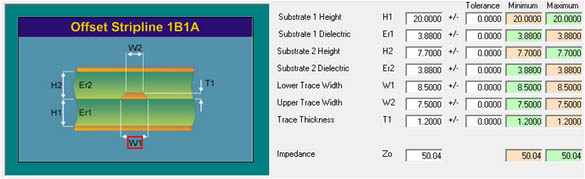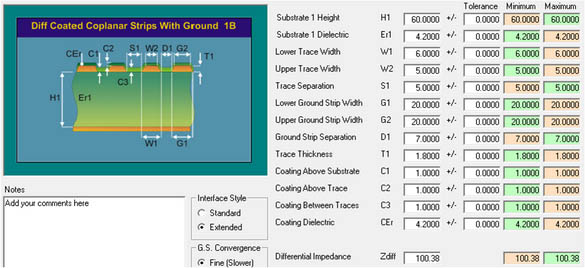전문 고주파 회로 기 판,고속 회로 기 판,IC 패 키 징 기 판,반도체 테스트 판,HDI 회로 기 판,소프트 하 드 결합 판,양면 다 층 판,PCB 디자인 및 PCBA 제조

### 빠 른 가격 획득.

sales@ipcb.com# PCB기술## PCB기술

PCB impedance calculation method

In the high speed PCB design process, PCB stack design and PCB impedance calculation are the first steps. PCB impedance calculation method is very mature, so the difference between different PCB software calculation is very small. This ipcber uses si9000 as an example.

PCB impedance calculation is relatively cumbersome, but we can sum up some experience values to help improve the calculation efficiency. For the commonly used FR4, 50ohm PCB microstrip line, the line width is generally equal to 2 times of the medium thickness; the line width of the 50 ohm stripline is equal to half of the total thickness of the medium between the two planes, which can help us quickly lock the range of PCB linewidth. Note that the calculated PCB linewidth is generally smaller than this value.

In addition to improving computational efficiency, we also need to improve PCB computing. Do you often encounter the inconsistency between the PCB impedance calculated by yourself and the PCB Factory? Some people will say that this has nothing to do with it. Let the PCB Factory adjust it directly. But will there be PCB Factory can not adjust, let you relax PCB impedance control? To do a good job in the product or everything in their own control is better.

The following points are put forward for your reference when designing stacked PCB impedance calculation:

1. PCB line width is preferred to be wide rather than thin. What does that mean? Because we know that there is a limit of fineness in PCB manufacturing process, and there is no limit to width. If the line width of PCB is narrowed in order to adjust the PCB impedance, it will be troublesome to increase the cost or relax the control of PCB impedance. Therefore, the relative width in calculation means that the target impedance is slightly lower. For example, the single line impedance is 50ohm. We can calculate it to 49ohm, and try not to calculate it to 51ohm.

2. There is a general trend. In our design, there may be multiple PCB impedance control targets, so the whole PCB impedance should be larger or smaller than 100 ohm and 90 ohm.

3. The residual copper rate and glue flow rate are considered. When one or both sides of the prepreg is etched with PCB circuit, the glue will fill in the etched gap during the pressing process, so that the adhesive thickness time between the two layers will be reduced. The smaller the residual copper rate, the more filled, the less remaining. Therefore, if the thickness of the two-layer prepreg you need is 5MIL, choose a slightly thicker prepreg according to the residual copper rate.

4. Specify glass cloth and glue content. Engineers who have seen PCB datasheets all know that the dielectric coefficient of different glass cloth, semi cured wafer or core board with different glue content is different. Even if it is almost the same height, it may be the difference of 3.5 and 4. This difference can cause the change of single line impedance about 3 ohm. In addition, the glass fiber effect is closely related to the size of the glass cloth window. If you have a design of 10Gbps or higher speed, and your lamination has no specified material, and the board factory uses a single sheet of 1080 PCB material, there may be signal integrity problems.

Of course, the calculation of residual copper rate and glue flow is inaccurate, the dielectric coefficient of PCB of new materials is sometimes inconsistent with the nominal value, and some PCB glass cloth factories do not prepare materials, etc., which will cause the design of lamination can not be realized or the delivery time is delayed. how? At the beginning of the design, let the plate factory design a stack according to our requirements and their experience, so that the ideal and realizable lamination can be obtained by more than a few rounds.

Last time, we talked about some "art of trade-off" between PCB impedance calculation and process planning, mainly to achieve the purpose of PCB impedance control, but also to ensure the convenience of process processing, as well as minimize the cost of PCB processing. Next, we will talk about the specific process of calculating PCB impedance with si9000.

How to calculate PCB impedance

For PCB impedance calculation, stack setting is a prerequisite. First of all, the specific stack information of a single board must be set first. The following is the PCB stacking information of a common eight layer PCB. Take this as an example to see some precautions for PCB impedance calculation.calculate PCB impedance

For the signal line, the implementation on the board can be divided into microstrip line and stripline. The difference between the two makes the structure of impedance calculation inconsistent. The following discusses the two common PCB impedance calculation cases.

a. PCB microstrip line

The characteristic of PCB microstrip line is that there is only one reference layer covered with green oil. The following is the specific parameter setting of single line (50 Ω) and differential line (100 Ω).PCB impedance  design considerations:

1. H1 is the medium thickness from the surface layer to the reference layer, excluding the copper thickness of the reference layer;

2. C1, C2 and C3 are the thickness of green oil. Generally, the thickness of green oil is about 0.5mil ~ 1mil, so it is good to keep the default value. The thickness has a slight impact on the impedance. This is also the reason why silk screen printing should not be placed on the impedance line as far as possible when processing text.

3. The thickness of T1 is generally the thickness of surface copper plus plating, and 1.8mil is the result of 0.5oz + plating.

4. Generally, W1 is the width of the line on the board. Since the processed line is trapezoidal, so W2

b. Stripline

A stripline is a wire that lies between two reference planes. The following is the specific parameter setting of single line (50 Ω) and differential line (100 Ω).

matters needing attention:

1. H1 is the thickness of core between the conductor and the reference layer, H2 is the thickness of PP between the conductor and the reference layer (considering the flow of PP); as shown in Figure 1, if the impedance line is in art03 layer, H1 is the dielectric thickness between gnd02 and art03, and H2 is the dielectric thickness between gnd04 and art03 plus copper thickness.

2. When the dielectric between ER1 and ER2 is different, the corresponding dielectric constant can be filled in.

3. The thickness of T1 is generally the thickness of inner layer copper; when the veneer is HDI board, it is necessary to pay attention to whether the inner layer is electroplated.

The above is the common calculation of PCB impedance line. However, due to the thicker board and fewer layers, the specific parameters of PCB impedance  line can not be calculated by using the above method. At this time, the coplanar impedance of PCB should be considered, as shown in the following figure:matters needing attention:

1. H1 is the thickness of the medium between the conductor and the near reference layer.

2. G1 and G2 are the width of adjoint ground. Generally, the larger the better.

3. D1 is the distance to the adjoining ground.

Question: after understanding the basic PCB impedance calculation, what factors are related to the PCB impedance  of signal lines on a single board, and what is their relationship (proportional or inverse)?